﻿ 小区开放对道路通行的影响——基于多层次模糊综合评价法 The Impact of Residential Area Opening on Road Traffic—Based on Multilevel Fuzzy Comprehensive Evaluation Method

Advances in Applied Mathematics
Vol. 08  No. 04 ( 2019 ), Article ID: 29995 , 14 pages
10.12677/AAM.2019.84094

The Impact of Residential Area Opening on Road Traffic

—Based on Multilevel Fuzzy Comprehensive Evaluation Method

Jiahui Zheng, Yixin Zhang, Jiangmin Wang

School of Mathematics and Data Science, Minjiang University, Fuzhou Fujian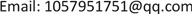Received: Apr. 6th, 2019; accepted: Apr. 21st, 2019; published: Apr. 28th, 2019ABSTRACT

Based on the Multilevel Fuzzy Comprehensive Evaluation Method, this paper chooses five evaluation indicators: road network structure, traffic capacity, road width and density, travel peak time and distance from the city center to analyze the impact of residential area opening on road traffic. Finally, it has become the focus of discussion to determine the impact of evaluation index on the road capacity around the residential area and whether the open residential area can achieve the goal of optimizing the road network structure, improving road capacity and improving traffic conditions.

Keywords:Open Community, Multilevel Fuzzy Comprehensive Evaluation, Network Structure, Traffic Capacity, Road Width and Density

——基于多层次模糊综合评价法Copyright © 2019 by authors and Hans Publishers Inc.1. 前言

2. 问题假设

1) 小区居民不对开放小区进行道路封闭。2) 小区的位置对各个权重影响不大。3) 不同类型小区车辆覆盖率基本一致。4) 小区开放的成本相差无几。5) 小区开放期间不受其他自然灾害影响。

3. 问题分析 

4. 小区开放对周边道路通行的影响的评价指标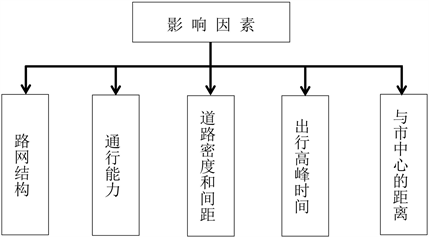Figure 1. Factors influencing traffic conditions in open residential areas

4.1. 路网结构

1) 路网的供需能力比

$\text{LOS}=\frac{T}{C}$ (1)

a) 路网容量：

b) 路网交通需求量：

$T={T}_{1}+{T}_{2}$ (2)

2) 利用路网时空饱和度指标

$\text{LOS}=\frac{n\cdot \mathrm{min}{P}_{dv}}{{P}_{s}}$ (3)

$n={n}_{1}+{n}_{2}$ (4)

${P}_{s}$ 为道路网车道的时空资源：

${P}_{s}=Lv\cdot t$ (5)

${P}_{dv}$ 为出行的时空资源消耗：

${P}_{dv}={h}_{y}\cdot t$ (6)

4.2. 通行能力

${N}_{p}=\frac{3600}{{t}_{i}}$ (7)

${N}_{m}={s}_{c}×{N}_{p}$ (8)Table 2. Classification coefficient of vehicle capacity

1) 根据交通流理论，反应 = 灵敏度 × 刺激。众所周知： $刺激={\stackrel{˙}{x}}_{i}\left(t\right)-{\stackrel{˙}{x}}_{i+1}\left(t\right)$$反应={\stackrel{¨}{x}}_{i+1}\left(t+T\right)$ ，且灵敏度分析公式为：

$灵敏度=\frac{a}{{\left[{x}_{i}\left(t\right)-{x}_{i+1}\left(t\right)\right]}^{m}}\left(a,m为待定参数\right)$

${\stackrel{¨}{x}}_{i+1}\left(t+T\right)=\left\{\frac{a}{{\left[{x}_{i}\left(t\right)-{x}_{i+1}\left(t\right)\right]}^{m}}\right\}\left({\stackrel{˙}{x}}_{i}\left(t\right)-{\stackrel{˙}{x}}_{i+1}\left(t\right)\right)$

$m=1$ 时， ${\stackrel{˙}{x}}_{i}\left(t+T\right)=a\mathrm{ln}\left[{x}_{i-1}\left(t\right)-{x}_{i}\left(t\right)\right]+{c}_{1}$

$m>1$ 时， ${\stackrel{˙}{x}}_{i}\left(t+T\right)={\left(1-m\right)}^{-1}a\mathrm{ln}\left[{x}_{i-1}\left(t\right)-{x}_{i}\left(t\right)\right]$

${c}_{2}=-{\left(1-m\right)}^{-1}a\mathrm{ln}{L}_{0}^{1-m}$

$m=1$ 时， ${\stackrel{˙}{x}}_{i}\left(t+T\right)=a\mathrm{ln}\left[\frac{{x}_{i-1}\left(t\right)-{x}_{i}\left(t\right)}{{L}_{0}}\right]$

$m>1$ 时， ${\stackrel{˙}{x}}_{i}\left(t+T\right)={\left(1-m\right)}^{-1}a\mathrm{ln}\left[{x}_{i-1}\left(t\right)-{x}_{i}\left(t\right)\right]$

$m=1$ ，则 $a=9$$\mu =9\cdot \frac{\mathrm{ln}{h}_{s}}{7}$ ，精度 $\theta =0.642$

$m=2$ ，则 $a=84$$\mu =98\cdot \left(\frac{1}{7}-\frac{1}{{h}_{s}}\right)$ ，精度 $\theta =0.991$

$m=3$ ，则 $a=730$$\mu =360\cdot \left(\frac{1}{{7}^{2}}-\frac{1}{{h}_{s}{}^{2}}\right)$ ，精度 $\theta =0.859$

$m=4$ ，则 $a=570$$\mu =1920\cdot \left(\frac{1}{{7}^{3}}-\frac{1}{{h}_{s}{}^{3}}\right)$ ，精度 $\theta =0.727$

$\mu =98\left(\frac{1}{7}-\frac{1}{{h}_{s}}\right)\left(\mu \le 9\text{\hspace{0.17em}}\text{m/s}\right)$ (9)

2) 道路通行能力 $\mu =98\left(\frac{1}{7}-\frac{1}{{h}_{s}}\right)\left(\mu \le 9\text{\hspace{0.17em}}\text{m/s}\right)$

${h}_{s}=\mu {h}_{t}$ ，故 $\mu =14-\frac{98}{{h}_{s}\mu }$ ，整理得 ${h}_{t}=\frac{98}{\mu \left(14-\mu \right)}$

${C}_{ap}=\mathrm{max}\frac{1}{{h}_{t}}=\mathrm{max}\frac{\mu \left(14-\mu \right)}{98}$ (10)Table 3. Headway time spacing and traffic capacity variable with vehicle speed

4.3. 道路密度和间距

1) 从交通的角度来看，道路网密度不能过稀和过密。

2) 道路网密度可以根据地区不同和交通管理控制方式而有所不同。

3) 但特殊地区可以另作考虑。

$\frac{1}{{d}_{i}}-\frac{1}{{d}_{i+1}}=\frac{{f}_{i}}{2000}$ (11)

5. 建立模型

5.1. 多层次模糊综合评价法

5.2. 模型建立步骤

$v=\left({v}_{1},{v}_{2},{v}_{3},{v}_{4},{v}_{5}\right)=\left(优,良,中,及格,不及格\right)$

$A=\left(\begin{array}{llll}{a}_{11}\hfill & {a}_{12}\hfill & \cdots \hfill & {a}_{1n}\hfill \\ {a}_{21}\hfill & {a}_{22}\hfill & \cdots \hfill & {a}_{2n}\hfill \\ ⋮\hfill & ⋮\hfill & \hfill & ⋮\hfill \\ {a}_{n1}\hfill & {a}_{n2}\hfill & \cdots \hfill & {a}_{nn}\hfill \end{array}\right)$

${W}_{i}=\frac{1}{n}-\frac{1}{2a}+\frac{1}{na}\underset{i=1}{\overset{n}{\sum }}{a}_{ij},i=1,2,\cdots ,n$(12)

$a$ 的范围为 $a\ge \frac{n-1}{2}$ ，表示 $a$ 越大，权重之差越小； $a$ 越小，权重之差越大，当时权重差异达到最大值  。

$R=\left({w}_{i1},{w}_{i2},\cdots ,{w}_{is}\right),i=1,2,\cdots ,s$

${G}_{i}=\left(\begin{array}{llll}{g}_{i11}\hfill & {g}_{i12}\hfill & \cdots \hfill & {g}_{i1m}\hfill \\ {g}_{i21}\hfill & {g}_{i22}\hfill & \cdots \hfill & {g}_{i2m}\hfill \\ ⋮\hfill & ⋮\hfill & \hfill & ⋮\hfill \\ {g}_{is1}\hfill & {g}_{is2}\hfill & \cdots \hfill & {g}_{ism}\hfill \end{array}\right)$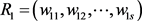5.3. 模型求解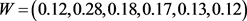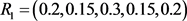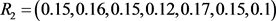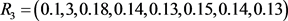Table 5. Scoring of traffic conditions affecting the opening of residential areas6. 各类型小区开放前后对道路通行的影响

6.1. 道路结构划分Table 6. Traffic conditions of various types of road structures (grades)Table 7. Traffic conditions of various types of road structures (scoring)

6.2. 通行能力Figure 2. Through road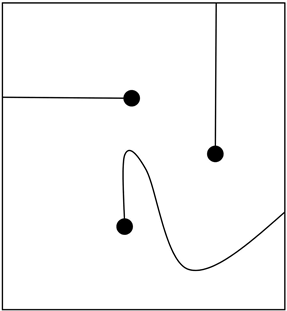Figure 3. End-to-end road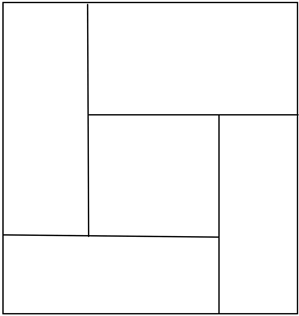Figure 4. Circular road

6.3. 道路密度和间距Table 9. Effects of road density and spacing on traffic in residential areas

6.4. 结合福州市某小区分析小区开放的结果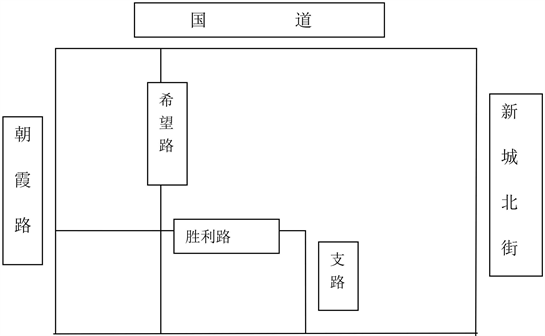Figure 5. Road layout before community opening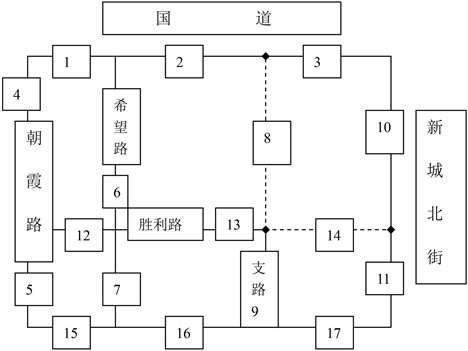Figure 6. Road layout after community opening

7. 关于小区开放的合理化建议

7.1. 小区开放原则

1) 将开放社区纳入执法机构的执法范围，依法收费，规范开放式小区道路交通秩序。

2) 小区开放时，将会有大量车辆进入小区，因此交通管理部门应将开放小区划入管理范围内。

3) 通过上述建立层次模糊分析法模型并对其结果分析得出，在影响小区道路通行的因素中也会产生相互影响。例如：小区路网结构与道路密度和间距之间相互制约的关系。因此，在小区道路的规划中，应根据小区的类型和小区的地理位置采取相应的措施，从而实现小区开放。Table 11. Closed residential quarters suitable for open road

7.2. 改善内容及依据

7.2.1. 改善内容

7.2.2. 改善措施依据

The Impact of Residential Area Opening on Road Traffic—Based on Multilevel Fuzzy Comprehensive Evaluation Method[J]. 应用数学进展, 2019, 08(04): 838-851. https://doi.org/10.12677/AAM.2019.84094

1. 1. 全国大学生数学建模竞赛组委会. 2016高教社杯全国大学生数学建模竞赛(CUMCM)题目B题[EB/OL]. http://www.mcm.edu.cn/, 2016-09-09.

2. 2. 姜启源, 谢金星, 叶俊. 数学模型[M]. 北京: 高等教育出版社, 2010.

3. 3. 杨淑娥. 多层次模糊综合评价法在课堂教学质量评价上的应用[J]. 徐州工程学院学报, 2008, 23(2): 72-77.

4. 4. 刘远才, 朱德滨. 城市小区道路交通与管理[J]. 林业建设, 2001(3): 29-33.

5. 5. 邹舒, 刘洪伟, 曲晓波. 多层次模糊综合评价法在评价教学质量中的应用[J]. 江苏技术师范学院学报(自然科学版), 2009, 15(3): 88-92.

6. 6. 杜艳青, 韩延成. 对外汉语教学的多层次模糊综合评价方法研究[J]. 郑州航空工业管理学院学报(社会科学版), 2006, 25(10): 169-171.